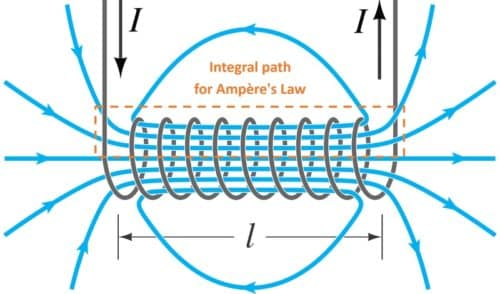# What Is Solenoid And How It Works?

This post was originally published on this site

Before we dive in deep into the principles of a  solenoid, let’s get a basic understanding of what an electromagnet is.

An electric current flowing through a coiled wire creates a magnetic field. When this wire is wound around a ferromagnetic or ferrimagnetic material, a magnet is created  known as an electromagnet. Since a magnetic field is produced as long as the current exits in the wire, the subsequent electromagnet has a temporary magnetic effect. When the current decreases to zero, there will be no magnetic effect.

## Introduction

Derived from two Greek words: Solen (pipe) and Eidos (coil), the solenoid is a type of an electromagnetic device that converts electrical energy into mechanical energy. It is generally made by tightly wounding wires in a helix shape around a piece of metal. Whenever an electric current passes through it, a magnetic field is created.

As previously stated, the power of the magnetic field depends on the electric current. Therefore, by varying the current as per our need, we can easily magnetise and demagnetise the electromagnet, enabling us to control the magnetic fields for different requirements.Courtesy: Wikipedia

## Working Principle

The solenoids work on electromagnetism and electromagnetic force. When a current passes through the coil a small amount of magnetic field is generated and in solenoid we use this electromagnetism to move plunger, armature as per our need.

The solenoid consists of a round cylindrical coil that has the number of turns of wire and a metal rod inside the coil that is free to move. So when the electric current is provided to the coil magnetic field is generated due to this magnetic field metal core or rod inside the coil attracted due to magnetic force of attraction.towards where the magnetic flux is high.

In this way we control the magnetic field of the coil by controlling the and use this as a mechanical movement of metal core named plunger.

B=µIN/L

B= Magnetic field

µ= Permeability

Where N is number of turns

I = current of coil

L= length of coil

Turns density n= N/L(Number of turns per unit length)

So from this formula we can see that if we want to increase the magnetic force produced in solenoid coil, then we can do it by increasing the number of turns and by increasing the current provided to the coil.

## Types Of Solenoid

### AC Laminated Solenoid

This type of solenoid has a very high initial attracting force and very closing time. It is made with very laminated metal or thin sheets that are individually insulated assembled.

### DC-C Frame Solenoid

As its name states it is a solenoid constructed in such a way that it has a Letter C like frame cover around the coil. This type are widely used in gaming machines.DC-C Frame Solenoid

### DC-D Frame Solenoid

As its name says, the solenoid coil is covered with two D frames of two sides of the coil. This types are generally used in AC Power.DC-D Frame Solenoid

### Linear Solenoid

This type of solenoid has a free movable steel or iron rod called plunger inside a round cylindrical shaped coil. The free Iron rod is allowed to move freely in or out od the cylindrical coil depending on the current applied.Linear Solenoid

### Rotary Solenoid

A rotary solenoid is a special type of solenoid where the magnetic force is converted into the rotation force or rotary motion. It consists of an armature core mounted on a flat disk.
When the current is provided

## Application

### Solenoid Valve

The solenoid valve is a simple device that uses the solenoid to control and regulate the flow of fluid. It has a coil with free movable plunger or Iron rod with a spring inside it. When we energise the coil due to magnetic attraction the plunger moves from its position and when we cut the power to coil the plunger comes back to its original position due to spring. We use this movement of plunger in controlling the fluid flow, when the plunger comes in the path of flowing fluid the flow stops due this obstacle. So this way we use the solenoid valve to control the flow of fluid.Solenoid Valve

### Solenoid Lock

Here we use the movement of solenoid plunger for locking and unlocking mechanism. These solenoid locks are widely used in electronic biometric , password based locks. These solenoid lock consist a moving metal strong plunger with solenoid coil when the coil get magnetised due to an electric field the plunger move to lock and unlock mechanism.Solenoid Lock